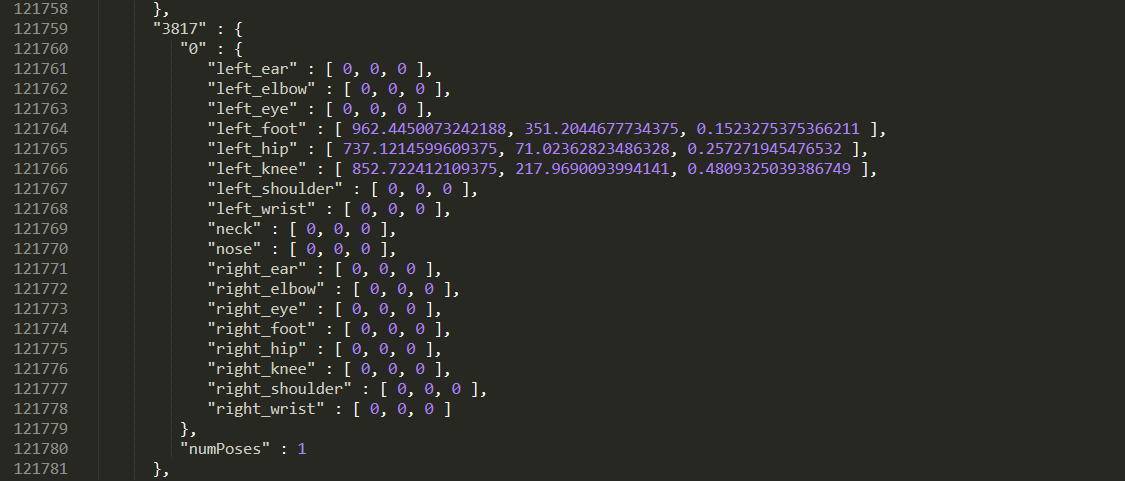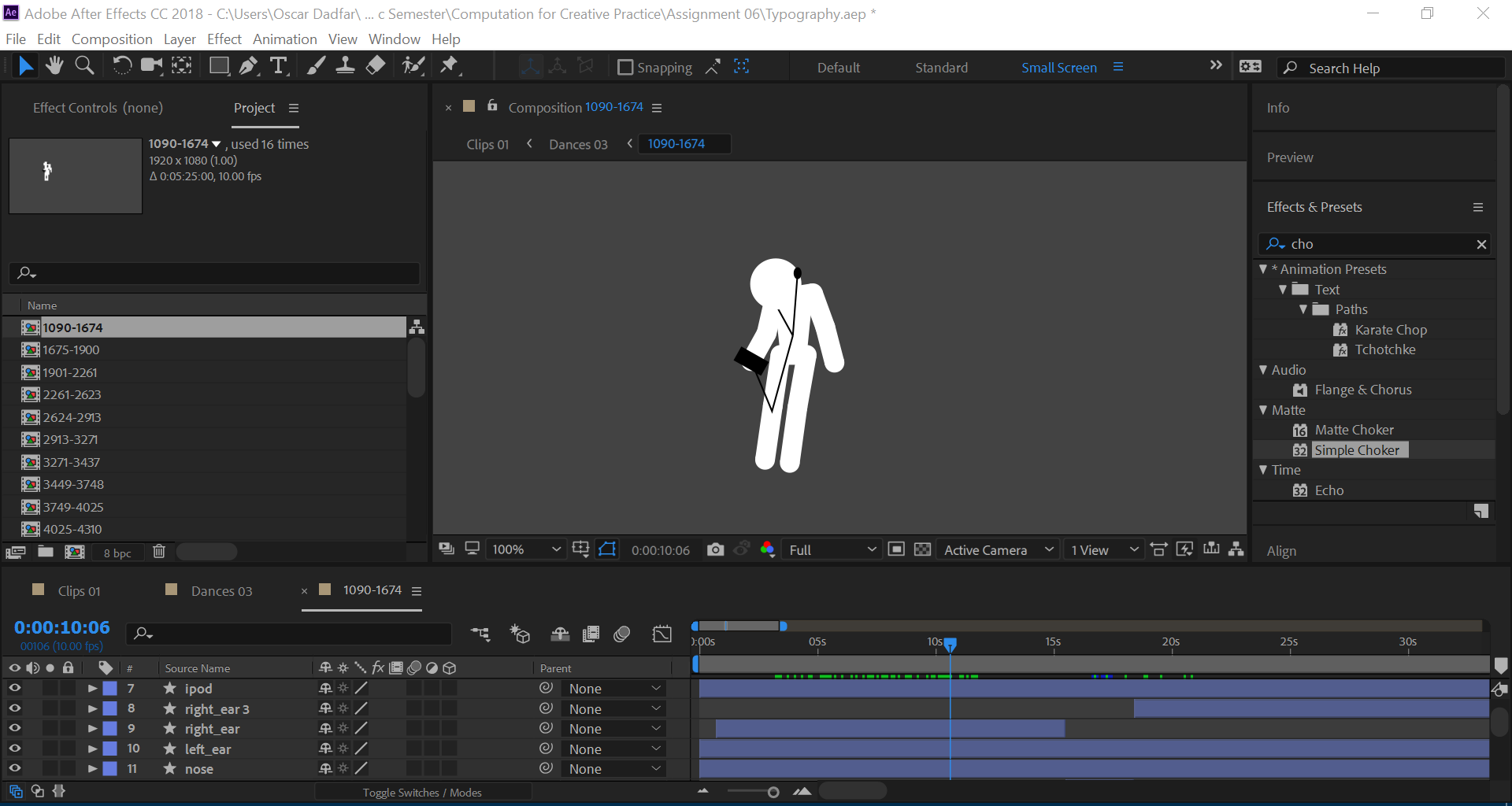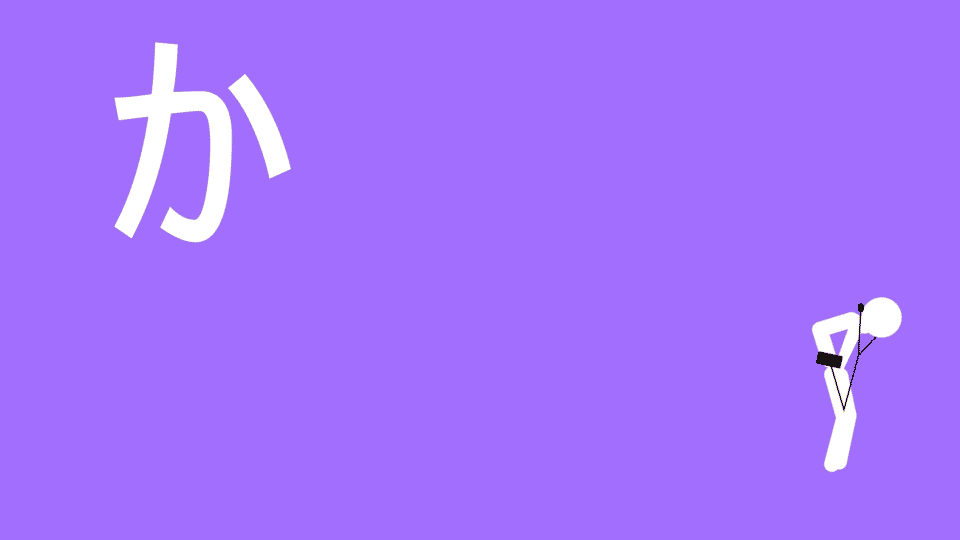# farcar – Mocap

Background

I have been working with After Effects for more than 5 years now, so when we were permitted to work on 2D platforms, I quickly made a google search for After Effects’ motion capture data transfer ability, particularly with bvh data files. The first result to come up was a \$40 pre-made After Effects script that converted bvh data to 2D skeletons.

https://aescripts.com/bvh-importer/

I could have applied for a micro-grant to purchase it. I could have called my parents asking for \$40. I could have used money from my own account. But I instead wrote my own script to do what the bvh importer could do, but for free.

Just at the time of this project, the Head TA was working on a JSON file containing motion capture data from the infamous Napoleon Dynamite Dance Scene. She was nice enough to give me the data, and I was quick to work on a script to convert that data into a readable format for After Effects.

This was my first time coding in After Effect’s scripting platform, ExtendScript, which is a .jsx format. Never heard of that format? Neither have I. .jsx is Adobe’s version of JavaScript preloaded with special Adobe commands listed in this .pdf reference sheet that was last updated following the release of CS6.

There was very little reference available on the language, meaning that not much help was at my disposal when I did Google searches for the style errors I came across. One really helpful script I did find was a JSON parsing script:

https://raw.githubusercontent.com/douglascrockford/JSON-js/master/json2.js

Methods

I began the process of drawing important body segments using ellipses in After Effects. Of the available body segments, I used the following data:

• left/right_wrist
• left/right_elbow
• left/right_shoulder
• neck
• left/right_waist
• left/right_knee
• left/right_foot

Once the joints were all drawn, I would proceed to generate vectors for the arms, legs, and remainder of the body. I did some sketching to find the best path for connecting the body with the minimal amount of vectors and shapesThe data reads (with slight modification):

• left_wrist -> left_elbow -> left_shoulder -> right_shoulder -> right_elbow -> right_wrist
• left_foot -> left_knee -> left_waist -> right_waist -> right_knee -> right_foot
• nose -> neck
• left_shoulder -> right_shoulder -> right_waist -> left_waist [rect group]

Once connecting the body with vectors, I had to find a way to connect the headphones too. Luckily, there was a data set for left/right_ear. I used this value to place headphones at these locations, and placed a phone at the position of left_wrist. Using the angle of the arm from left_elbow to left_wrist, I had the phone rotate accordingly. I then generated a line coming from the left_wrist to 50 pixels below the neck where the headphone would split. Taking that point below the neck, I then generated two additional vectors from that point to each ear to make headphones. As a final tweak, I added a point on the vector leading from the phone to the point below the neck to make the wire look as if it is under the influence of gravity. The height of the dip was dependent on the distance between the left_wrist and the neck.

From here, I had working models that could be generated from the JSON data. The original JSON contained nearly 5000 frames, too much for my laptop to handle. To cope with this, I separated the frames into intervals of 400, copying each batch of 400 frames into a separate JSON for processing. I was left with 11 compositions of data, each with ~20 seconds of animation. Though, nearly half of the data was unusable. This was because the data contained in the JSON was unable to identify certain body parts during certain frames, leaving 0’s in its trail.I could only salvage frames that had non-zero values, or else a body part would fly off-screen.

After going through all the compositions, I was left with 14 unique dance cycles averaging 5 seconds each. I did some additional layering edits with the cycles before I went into editing. The reason I wanted to use motion capture in After Effects was to be able to animate text over the dancing figures.Results

Info about audio, softwares, and additional production details can be found in the video description.Code

```#include "json2.js"   var thisComp = app.project.activeItem;   var script_file = File(\$.fileName); var script_file_path = script_file.path;   var file_to_read = File(script_file_path + "/output2.json");   var content; if(file_to_read !== false){ file_to_read.open('r'); response = file_to_read.read(); content = JSON.parse(response); clear_layers(); animate(content); file_to_read.close(); }   function clear_layers() { var len = thisComp.numLayers; for(var i = 0; i < len; i++) thisComp.layer(1).remove(); }   function animate(content) { var fps = 20; var parts = ["left_wrist", "left_elbow", "left_shoulder", "right_shoulder", "right_elbow", "right_wrist", "left_foot", "left_knee", "left_hip", "right_hip", "right_knee", "right_foot", "nose", "neck"]; var joints = ["left_wrist_elbow", "left_elbow_shoulder", "left_right_shoulder", "right_elbow_shoulder", "right_wrist_elbow", "space", "left_foot_knee", "left_knee_hip", "left_right_hip", "right_knee_hip", "right_foot_knee", "space2", "nose_neck"]; var partsLen = parts.length;   new_rectangle("body");   for(var i = 0; i < joints.length; i++) new_line(joints[i]);   for(var i = 0; i < partsLen; i++) new_ellipse(parts[i]);   new_ellipse("left_ear"); new_ellipse("right_ear");   new_rectangle("ipod");   new_line("cord"); new_line("left_cord"); new_line("right_cord");   var start = 465; var end = 972; var step = 1; var totalStep = 0;   var right_alive_x = 0; var right_alive_y = 0;   for(var h = start; h < end; h++) { step = content[String(h)]["numPoses"]; for(var i = 0; i < step; i++) { if(content[String(h)][String(i)] !== undefined) {   var x1 = content[String(h)][String(i)]["left_shoulder"]; var y1 = content[String(h)][String(i)]["left_shoulder"]; var x2 = content[String(h)][String(i)]["right_shoulder"]; var y2 = content[String(h)][String(i)]["right_shoulder"]; var x3 = content[String(h)][String(i)]["left_hip"]; var y3 = content[String(h)][String(i)]["left_hip"]; var x4 = content[String(h)][String(i)]["right_hip"]; var y4 = content[String(h)][String(i)]["right_hip"]; var shape = new Shape(); shape.vertices = [[x1-960,y1-540],[x2-960,y2-540], [x3-960,y3-540],[x4-960,y4-540]]; shape.closed = true;   var shapeGroup = thisComp.layer("body").property("Contents"); shapeGroup.property(1).property("ADBE Vector Shape").setValueAtTime( (totalStep)/fps, shape);       for(var j = 0; j < partsLen; j++) {   var x = content[String(h)][String(i)][parts[j]]; var y = content[String(h)][String(i)][parts[j]]; thisComp.layer(parts[j]).position.setValueAtTime( (totalStep)/fps, [x, y]);   if(j < partsLen-1 && j !== 5 && j !==11) {   var a = content[String(h)][String(i)][parts[j+1]]; var b = content[String(h)][String(i)][parts[j+1]];   var shape = new Shape(); shape.vertices = [[x-960,y-540],[a-960,b-540]]; shape.closed = false; var shapeGroup = thisComp.layer(joints[j]).property("Contents"); shapeGroup.property(1).property("ADBE Vector Shape").setValueAtTime( (totalStep)/fps, shape); } }   var x = content[String(h)][String(i)]["left_ear"]; var y = content[String(h)][String(i)]["left_ear"]; thisComp.layer("left_ear").position.setValueAtTime( (totalStep)/fps, [x-10, y]);   var x = content[String(h)][String(i)]["right_ear"]; var y = content[String(h)][String(i)]["right_ear"]; thisComp.layer("right_ear").position.setValueAtTime( (totalStep)/fps, [x+10, y]);     var x1 = content[String(h)][String(i)]["left_wrist"]; var y1 = content[String(h)][String(i)]["left_wrist"]; var x2 = content[String(h)][String(i)]["neck"]; var y2 = content[String(h)][String(i)]["neck"]+50; var shape = new Shape(); shape.vertices = [[x1-960,y1-540],[(x1+x2)/2-960,y1-530 + 0.1*(x1+x2)/2],[x2-960,y2-540]]; shape.closed = false; var shapeGroup = thisComp.layer("cord").property("Contents"); shapeGroup.property(1).property("ADBE Vector Shape").setValueAtTime( (totalStep)/fps, shape);     var x3_l = content[String(h)][String(i)]["left_ear"]; var y3_l = content[String(h)][String(i)]["left_ear"]; var shape = new Shape(); if(content[String(h)][String(i)]["left_ear"] > 1) { shape.vertices = [[x2-960,y2-540],[x3_l-970,y3_l-540]]; } else { x3_l = content[String(h)][String(i)]["nose"]-25; y3_l = content[String(h)][String(i)]["nose"]-10; shape.vertices = [[x2-960,y2-540],[x3_l-955,y3_l-540]]; } shape.closed = false; var shapeGroup = thisComp.layer("left_cord").property("Contents"); shapeGroup.property(1).property("ADBE Vector Shape").setValueAtTime( (totalStep)/fps, shape);     var x3_r = content[String(h)][String(i)]["right_ear"]; var y3_r = content[String(h)][String(i)]["right_ear"]; var shape = new Shape(); if(content[String(h)][String(i)]["right_ear"] > 1) shape.vertices = [[x2-960,y2-540],[x3_r-950,y3_r-540]]; else { x3_r = content[String(h)][String(i)]["nose"]+25; y3_r = content[String(h)][String(i)]["nose"]-10; shape.vertices = [[x2-960,y2-540],[x3_r-955,y3_r-540]]; } shape.closed = false; var shapeGroup = thisComp.layer("right_cord").property("Contents"); shapeGroup.property(1).property("ADBE Vector Shape").setValueAtTime( (totalStep)/fps, shape);     var x = content[String(h)][String(i)]["left_wrist"]; var y = content[String(h)][String(i)]["left_wrist"]; var shapeGroup = thisComp.layer("ipod").property("Contents"); thisComp.layer("ipod").position.setValueAtTime( (totalStep)/fps, [x, y]);   var x2 = content[String(h)][String(i)]["left_elbow"]; var y3 = content[String(h)][String(i)]["left_elbow"]; var dist = Math.sqrt( (y2-y)*(y2-y) + (x2-x)*(x2-x) ); var dist_y = Math.abs(y2 - y); var r = (90/3.14)*Math.asin(dist_y/dist)+90;   thisComp.layer("ipod").rotation.setValueAtTime( (totalStep)/fps, r); } totalStep++; } }   thisComp.layer("space").remove(); thisComp.layer("space2").remove(); }   function new_ellipse(name) { var shapeLayer = thisComp.layers.addShape(); var shapeGroup = shapeLayer.property("Contents").addProperty("ADBE Vector Group"); shapeGroup.property("Contents").addProperty("ADBE Vector Shape - Ellipse"); var fill = shapeGroup.property("Contents").addProperty("ADBE Vector Graphic - Fill"); if(name == "left_ear" || name == "right_ear") { fill.property("Color").setValue([0,0,0]); shapeLayer.scale.setValue([10,15]); } else { fill.property("Color").setValue([255,255,255]); if(name == "nose") shapeLayer.scale.setValue([65,65]); else shapeLayer.scale.setValue([25,25]); } shapeLayer.name = name; }   function new_rectangle(name) { var shapeLayer = thisComp.layers.addShape(); var shapeGroup = shapeLayer.property("ADBE Root Vectors Group"); shapeGroup.addProperty("ADBE Vector Shape - Group"); var fill = shapeGroup.addProperty("ADBE Vector Graphic - Fill");   if(name == "ipod") { fill.property("Color").setValue([0,0,0]);   var x_scl = 10; var y_scl = 20;   var shape = new Shape(); shape.vertices = [[-x_scl,-y_scl],[x_scl,-y_scl],[x_scl,y_scl],[-x_scl,y_scl]]; shape.closed = true; } else { fill.property("Color").setValue([255,255,255]);   var shape = new Shape(); shape.vertices = [[0,0],[0,0],[0,0],[0,0]]; shape.closed = true; }   shapeGroup.property(1).property("ADBE Vector Shape").setValue(shape); shapeLayer.name = name; }   function new_line(name) { var shapeLayer = thisComp.layers.addShape(); var shapeGroup = shapeLayer.property("ADBE Root Vectors Group"); shapeGroup.addProperty("ADBE Vector Shape - Group"); var stroke = shapeGroup.addProperty("ADBE Vector Graphic - Stroke"); if(name == "cord" || name == "left_cord" || name == "right_cord") { stroke.property("Stroke Width").setValue(); stroke.property("Color").setValue([0,0,0]); } else { stroke.property("Stroke Width").setValue(); stroke.property("Color").setValue([255,255,255]); }     var shape = new Shape(); shape.vertices = [[0,0],[0,0]]; shape.closed = false;   shapeGroup.property(1).property("ADBE Vector Shape").setValue(shape);   shapeLayer.name = name; }```